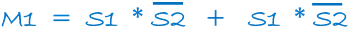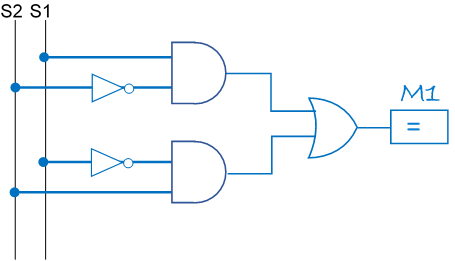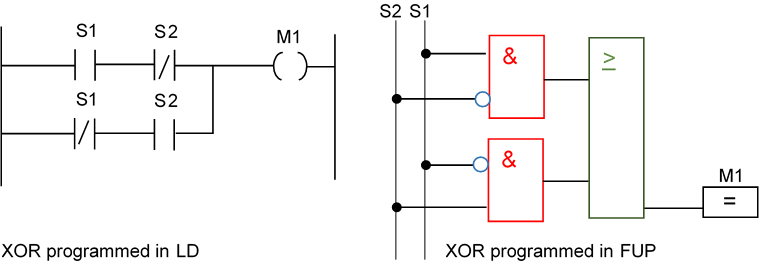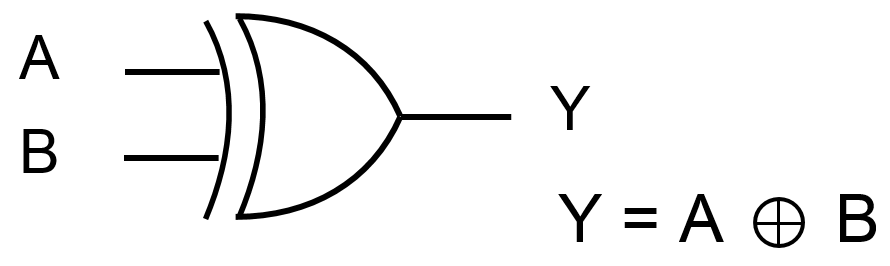### Circuit design using a simple example

You receive a plant description from your customer and must develop a PLC program for this purpose. Which work steps are necessary for this? This will be shown by means of a simple example.

Example: It should be possible to switch on a motor M1 from two control points with the latching switches S1 and S2. The motor should start when either only S1 or only S2 is switched on.

Step 1:   Create a Truth tableTruth table

Step 2:   Create a Boolean equation from the Truth table

Only the cases in which the output has the logical state '1' are interesting here! The complete Boolean equation is:Boolean equation

Step 3:   Translate the logic equation into a Logic circuitLogic circuit

Using a  PLC you might program in LD (Ladder Diagram) or FUP (Function plan). Here the solution:Learning PLC - XOR in LD and FUP

Note: This logic, i.e. that the output only switches when only one input has High-signal at any one time, is frequently used in automation technology. Therefore, it has its own designation and symbol:XOR, Exclusive-Or